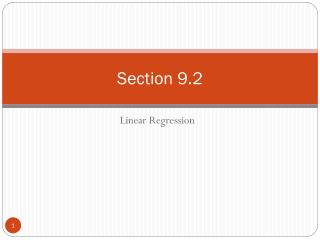DownloadDownload PresentationSection 9.2

# Section 9.2

Télécharger la présentation## Section 9.2

- - - - - - - - - - - - - - - - - - - - - - - - - - - E N D - - - - - - - - - - - - - - - - - - - - - - - - - - -
##### Presentation Transcript

1. Section 9.2 Linear Regression

2. Section 9.2 Objectives • Find the equation of a regression line • Predict y-values using a regression equation

3. Regression lines • After verifying that the linear correlation between two variables is significant, next we determine the equation of the line that best models the data (regression line). • Can be used to predict the value of y for a given value of x. y x

4. Residuals Residual • The difference between the observed y-value and the predicted y-value for a given x-value on the line. For a given x-value, di = (observed y-value) – (predicted y-value) Observed y-value y d6{ d4{ }d5 d3{ }d2 Predicted y-value }d1 x

5. Regression Line Regression line(line of best fit) • The line for which the sum of the squares of the residuals is a minimum. • The equation of a regression line for an independent variable x and a dependent variable y is ŷ = mx + b y-intercept Predicted y-value for a given x-value Slope

6. The Equation of a Regression Line • ŷ = mx + b where • is the mean of the y-values in the data • is the mean of the x-values in the data • The regression line always passes through the point

7. Example: Finding the Equation of a Regression Line Find the equation of the regression line for the advertising expenditures and company sales data.

8. Solution: Finding the Equation of a Regression Line Recall from section 9.1: 540 5.76 50,625 294.4 2.56 33,856 440 4 48,400 624 6.76 57,600 252 1.96 32,400 294.4 2.56 33,856 372 4 34,596 473 4.84 46,225 Σx = 15.8 Σy = 1634 Σxy = 3289.8 Σx2 = 32.44 Σy2 = 337,558

9. Solution: Finding the Equation of a Regression Line Σx = 15.8 Σy = 1634 Σxy = 3289.8 Σx2 = 32.44 Σy2 = 337,558 Equation of the regression line

10. Solution: Finding the Equation of a Regression Line • To sketch the regression line, use any two x-values within the range of the data and calculate the corresponding y-values from the regression line. y Company sales (in thousands of dollars) x Advertising expenses (in thousands of dollars)

11. Example: Using Technology to Find a Regression Equation Use a technology tool to find the equation of the regression line for the Old Faithful data.

12. Example: Predicting y-Values Using Regression Equations The regression equation for the advertising expenses (in thousands of dollars) and company sales (in thousands of dollars) data is ŷ = 50.729x + 104.061. Use this equation to predict the expected company sales for the following advertising expenses. (Recall from section 9.1 that x and y have a significant linear correlation.) • 1.5 thousand dollars • 1.8 thousand dollars • 2.5 thousand dollars

13. Solution: Predicting y-Values Using Regression Equations ŷ = 50.729x + 104.061 • 1.5 thousand dollars ŷ =50.729(1.5) + 104.061 ≈ 180.155 When the advertising expenses are \$1500, the company sales are about \$180,155. 1.8 thousand dollars ŷ =50.729(1.8) + 104.061 ≈ 195.373 When the advertising expenses are \$1800, the company sales are about \$195,373.

14. Solution: Predicting y-Values Using Regression Equations • 2.5 thousand dollars ŷ =50.729(2.5) + 104.061 ≈ 230.884 When the advertising expenses are \$2500, the company sales are about \$230,884. Prediction values are meaningful only for x-values in (or close to) the range of the data. The x-values in the original data set range from 1.4 to 2.6. So, it would not be appropriate to use the regression line to predict company sales for advertising expenditures such as 0.5 (\$500) or 5.0 (\$5000).

15. Section 9.2 Summary • Found the equation of a regression line • Predicted y-values using a regression equation